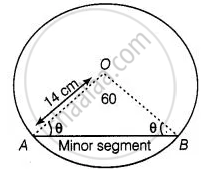# On a square handkerchief, nine circular designs each of radius 7 cm are made (see figure). Find the area of the remaining portion of the handkerchief. - Mathematics

Sum

Find the area of the minor segment of a circle of radius 14 cm, when the angle of the corresponding sector is 60°.

#### SolutionGiven that, radius of circle (r) = 14 cm

And angle of the corresponding sector i.e., central angle (θ) = 60°

Since, in ΔAOB, OA = OB = Radius of circle i.e., ΔAOB is isosceles.

⇒ ∠AOB = ∠OBA = θ

Now, In ΔAOB,

∠AOB + ∠OAB = ∠OBA = 180°   ......[Since, sum of interior angles of any triangle is 180°]

⇒ 60° + θ + θ = 180°

⇒ 2θ = 120°

⇒ θ = 60°

i.e. ∠OAB = ∠OBA = 60° = ∠AOB

Since, all angles of ΔAOB are equal to 60° i.e., ΔAOB ia an equilateral triangle.

Also, OA = OB = AB = 14 cm

So, Area of ΔOAB = sqrt(3)/4 (side)2

=  sqrt(3)/4 xx (14)^2   ......[∵ Area of an equilateral triangle = sqrt(3)/4 (sides)2]

= sqrt(3)/4 xx 196

= 49sqrt(3) cm2

Concept: Areas of Sector and Segment of a Circle
Is there an error in this question or solution?

#### APPEARS IN

NCERT Mathematics Exemplar Class 10
Chapter 11 Area Related To Circles
Exercise 11.3 | Q 10 | Page 127
Share